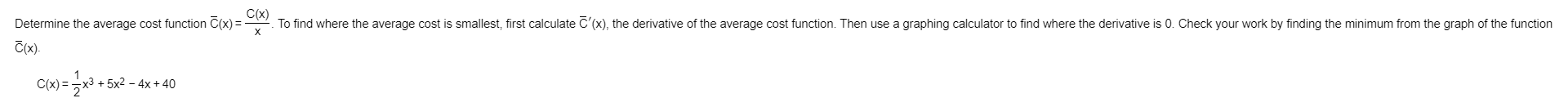# To find where the average cost is smallest, first calculate C'(x), the derivative of the average cost function. Then use a graphing calculator to find where the derivative is 0. Check your work by finding the minimum from the graph of the functionC(x)Determine the average cost function C(x)C(x)x)=x3 +5x2 - 4x+ 40

Question
383 views

Please solve for all parts of questionhelp_outlineImage TranscriptioncloseTo find where the average cost is smallest, first calculate C'(x), the derivative of the average cost function. Then use a graphing calculator to find where the derivative is 0. Check your work by finding the minimum from the graph of the function C(x) Determine the average cost function C(x) C(x) x)=x3 +5x2 - 4x+ 40 fullscreen
check_circle

Given,

...

### Want to see the full answer?

See Solution

#### Want to see this answer and more?

Solutions are written by subject experts who are available 24/7. Questions are typically answered within 1 hour.*

See Solution
*Response times may vary by subject and question.
Tagged in

### Calculus